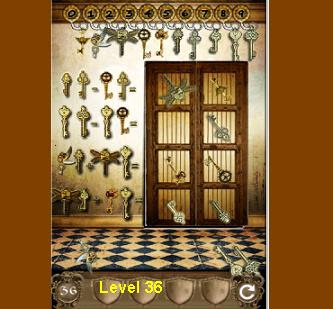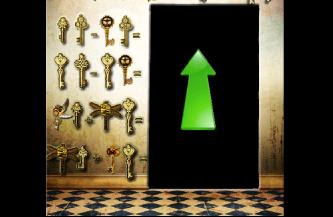100 Gates Level 36

New update has arrived hour ago. This is guide for 100 gates level 36. how to pass the game? it's not so hard to beat this puzzle. Check out our hints below."100 gates 36"

In this stage you will see many keys. Sure, you will ask question : where is the code? because in previous level we will always see code above the door, but know there is no code or symbol as clue to solve "100 gates level 36" game?

Don't worry because the code is still there in the place as always be, which is above the door. There are 10 keys starting from number 0 in the left to number 9 in the right. Please remember the shape of each key.

Now move to the equation on the left side of door,
change key with its number, then you will find for100 gates level 36

• top row equation : 56 - 36 = 20 so this is answer for top 2 rectangles on door
• next row is 89 - 83 = 6 that's why in this row there is only one rectangle
• next row is 15 + 28 = 43
• bottom row is 27 + 48 = 75 this is the last game answer

Now fill the rectangles on the door with the correct key from the ground, Simply tap and then slide the key to the rectangles on door

That's game answer /solution for 100 gates level 36. Go to next guide here